Writing Fractions as Decimals worksheet

Chapter 8 Class 6 Decimals
Serial order wise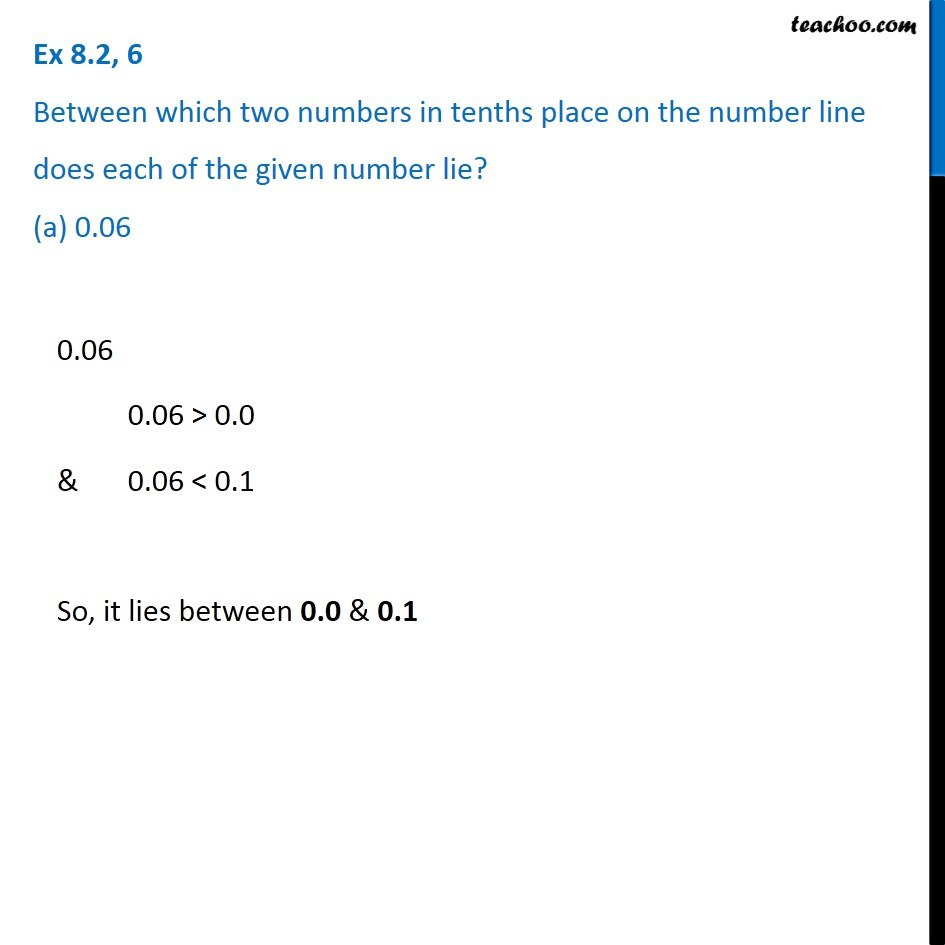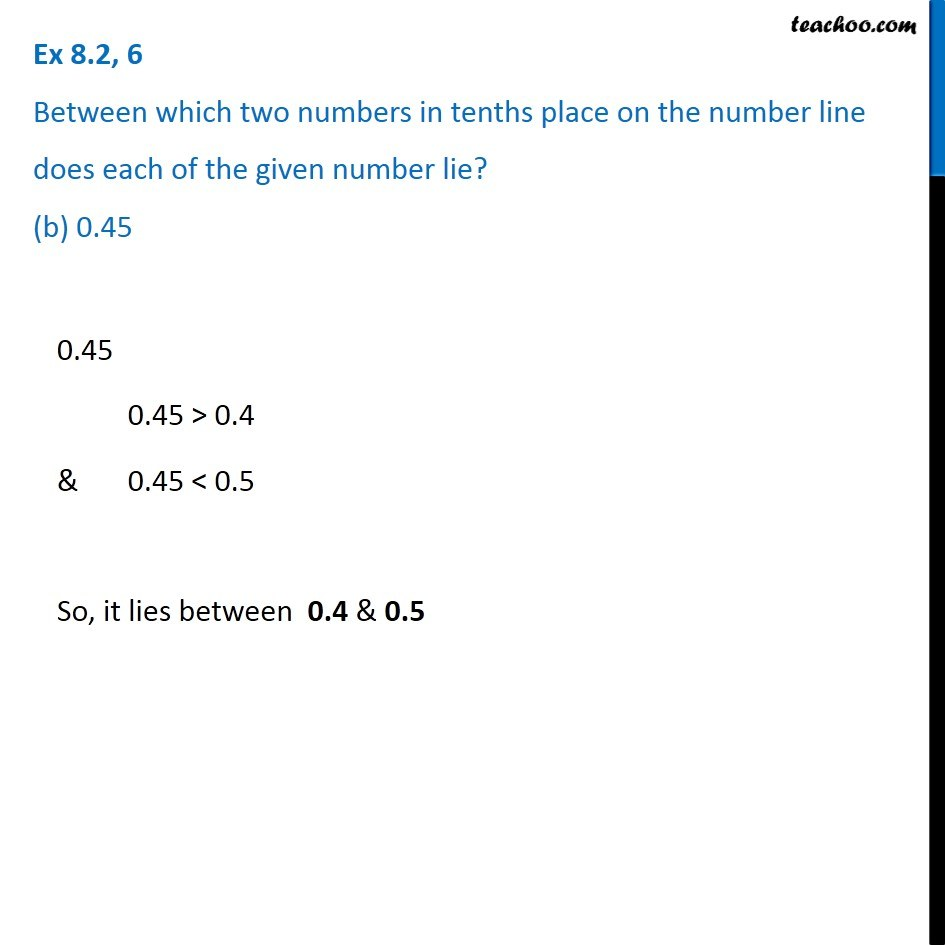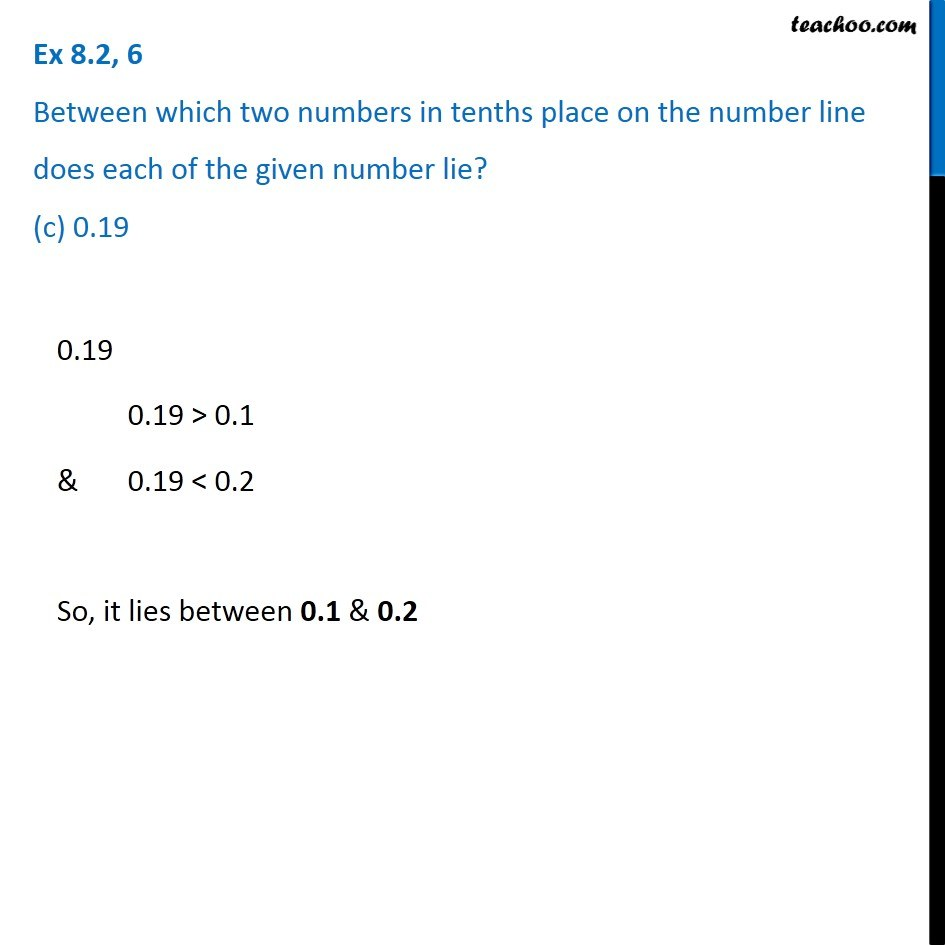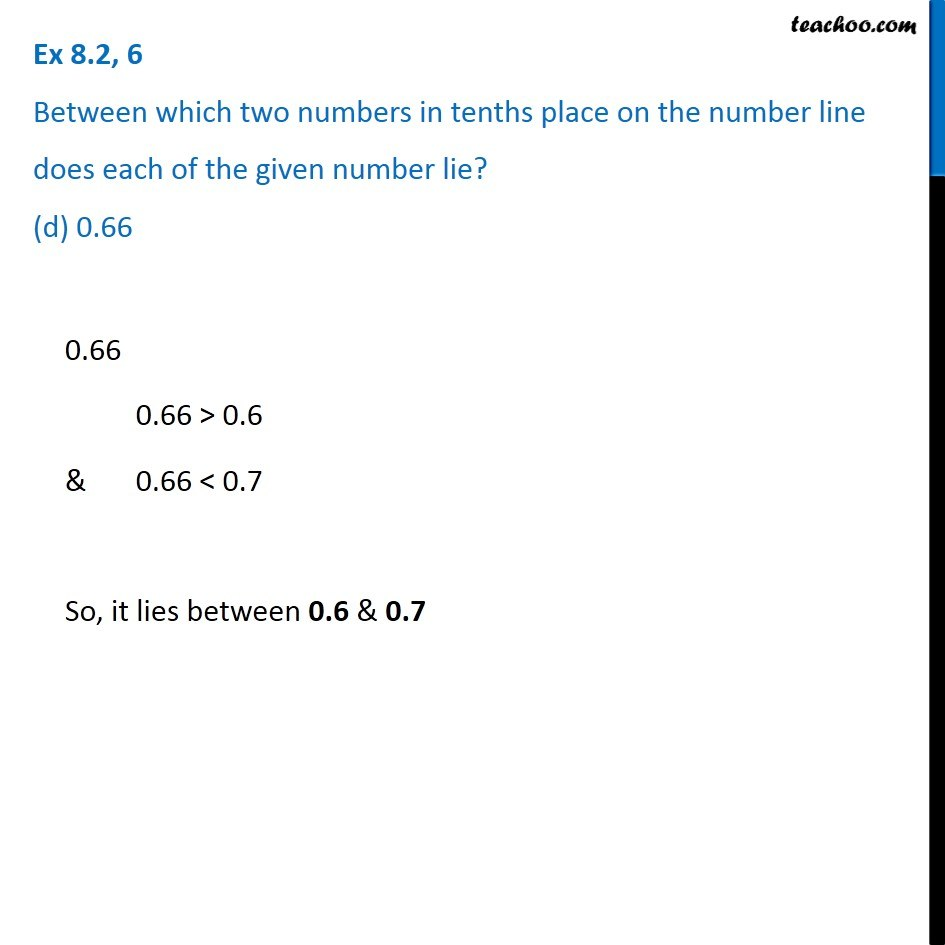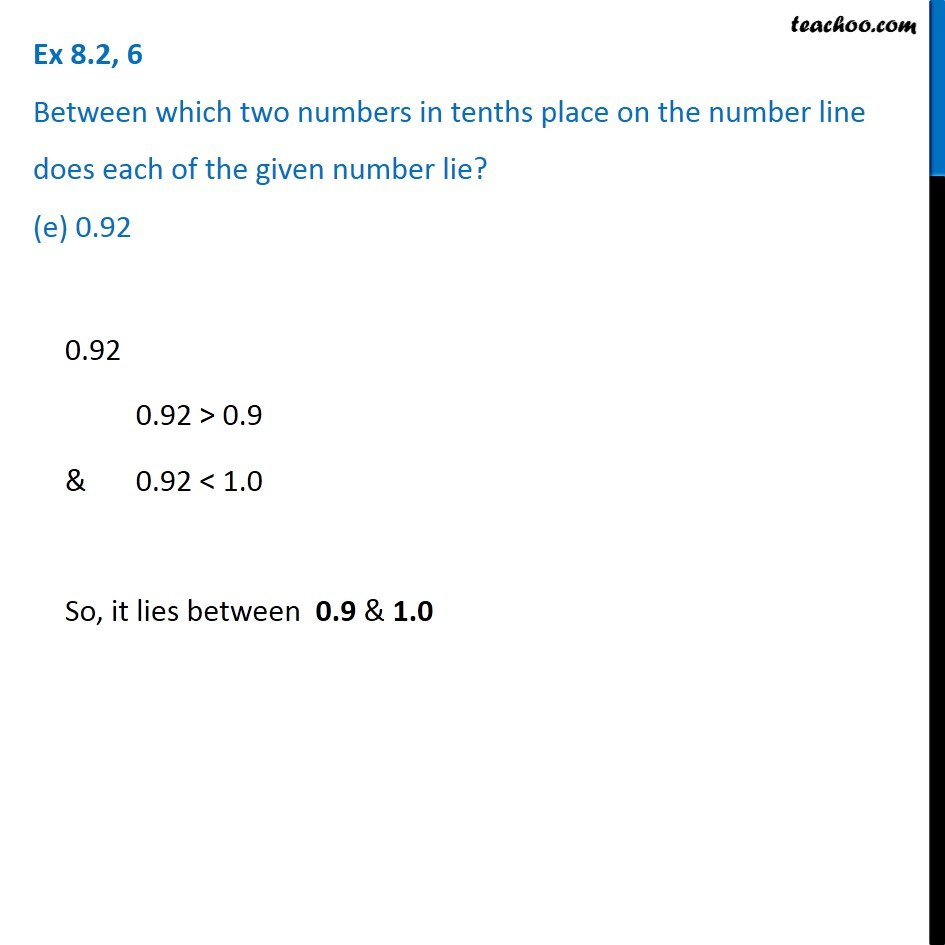Learn in your speed, with individual attention - Teachoo Maths 1-on-1 Class

### Transcript

Question 6 Between which two numbers in tenths place on the number line does each of the given number lie? (a) 0.06 0.06 0.06 > 0.0 & 0.06 < 0.1 So, it lies between 0.0 & 0.1 Question 6 Between which two numbers in tenths place on the number line does each of the given number lie? (b) 0.45 0.45 0.45 > 0.4 & 0.45 < 0.5 So, it lies between 0.4 & 0.5 Question 6 Between which two numbers in tenths place on the number line does each of the given number lie? (c) 0.19 0.19 0.19 > 0.1 & 0.19 < 0.2 So, it lies between 0.1 & 0.2 Question 6 Between which two numbers in tenths place on the number line does each of the given number lie? (d) 0.66 0.66 0.66 > 0.6 & 0.66 < 0.7 So, it lies between 0.6 & 0.7 Question 6 Between which two numbers in tenths place on the number line does each of the given number lie? (e) 0.92 0.92 0.92 > 0.9 & 0.92 < 1.0 So, it lies between 0.9 & 1.0 Question 6 Between which two numbers in tenths place on the number line does each of the given number lie? (f) 0.57 0.57 0.57 > 0.5 & 0.57 < 0.6 So, it lies between 0.5 & 0.6